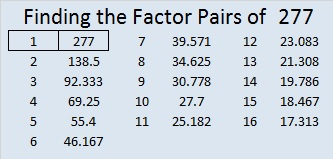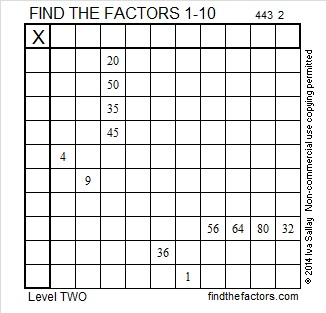# 277 and Level 2

• 277 is a prime number.
• Prime factorization: 277 is prime.
• The exponent of prime number 277 is 1. Adding 1 to that exponent we get (1 + 1) = 2. Therefore 277 has exactly 2 factors.
• Factors of 277: 1, 277
• Factor pairs: 277 = 1 x 277
• 277 has no square factors that allow its square root to be simplified. √277 ≈ 16.643How do we know that 277 is a prime number? If 277 were not a prime number, then it would be divisible by at least one prime number less than or equal to √277 ≈ 16.643. Since 277 cannot be divided evenly by 2, 3, 5, 7, 11, or 13, we know that 277 is a prime number.

What can a level 2 puzzle do? Sometimes the “impossible” as this tweet suggests: